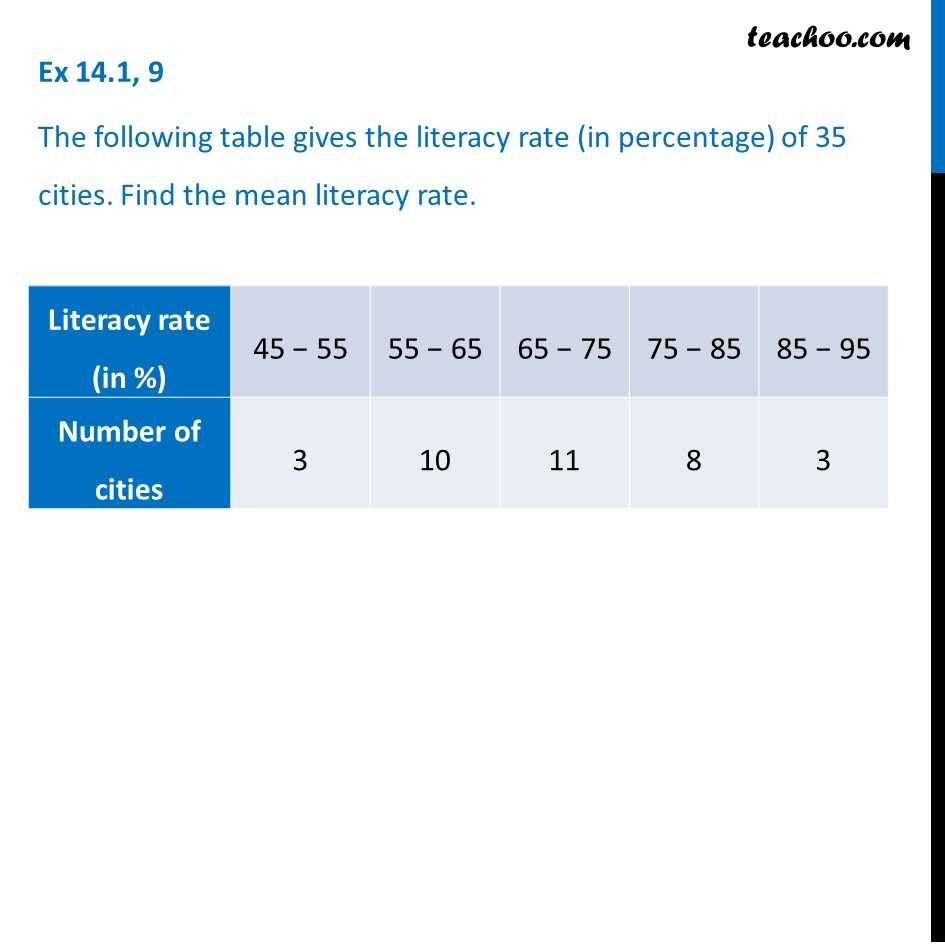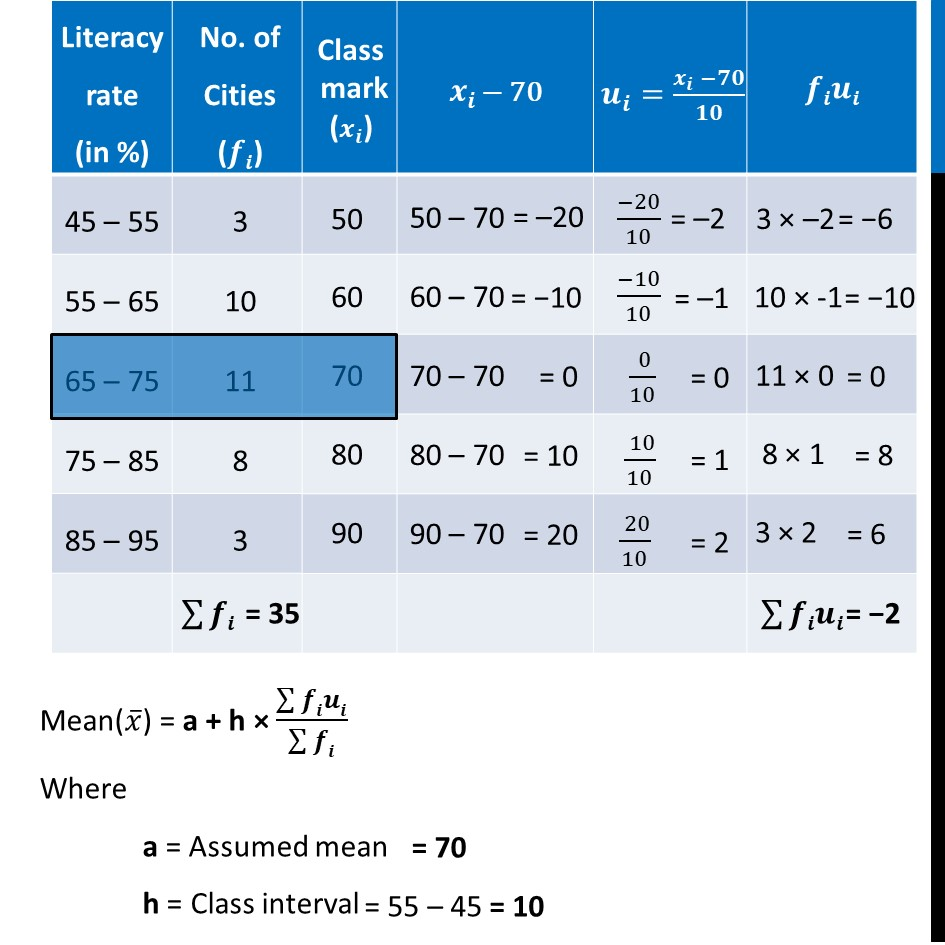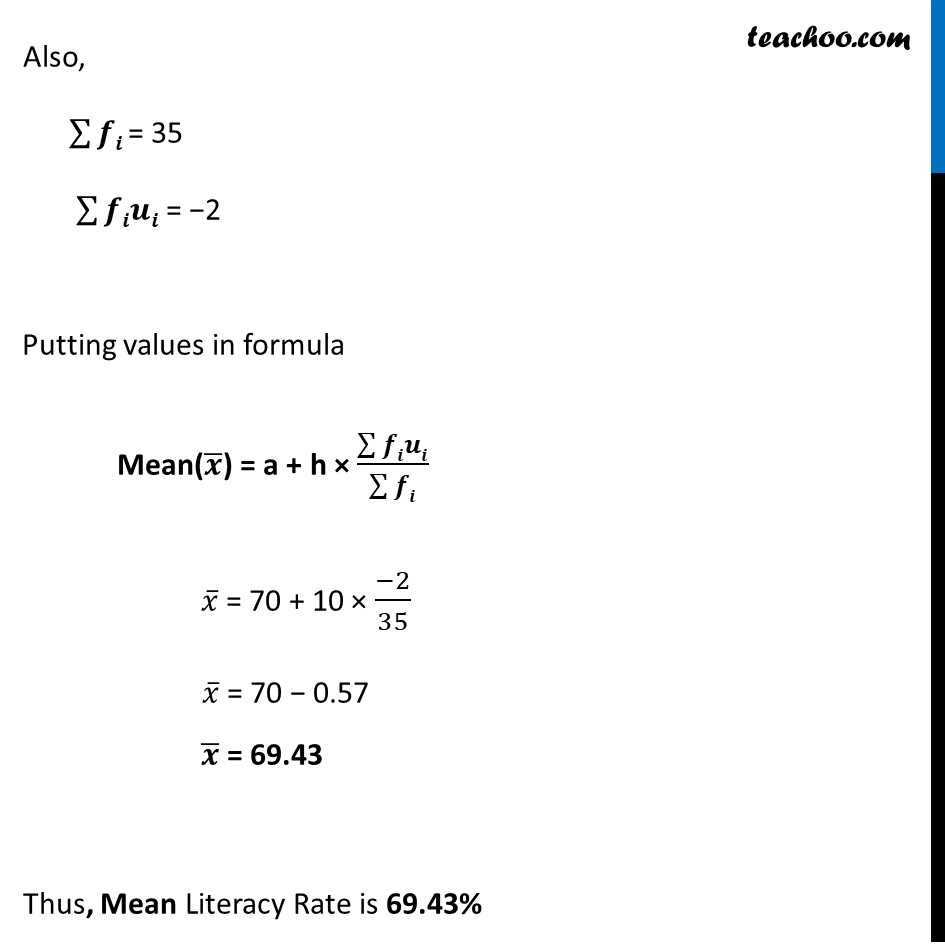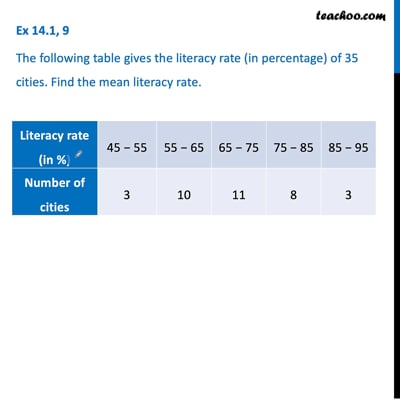Ex 14.1

Chapter 14 Class 10 Statistics
Serial order wiseThis video is only available for Teachoo black users

Maths Crash Course - Live lectures + all videos + Real time Doubt solving!

### Transcript

Ex 14.1, 9 The following table gives the literacy rate (in percentage) of 35 cities. Find the mean literacy rate. Mean(𝑥 ̅) = a + h × (∑▒𝒇𝒊𝒖𝒊)/(∑▒𝒇𝒊) Where a = Assumed mean h = Class interval = 70 = 55 – 45 = 10 Also, ∑▒𝒇𝒊 = 35 ∑▒𝒇𝒊𝒖𝒊 = −2 Putting values in formula Mean(𝒙 ̅) = a + h × (∑▒𝒇𝒊𝒖𝒊)/(∑▒𝒇𝒊) 𝑥 ̅ = 70 + 10 × (−2)/35 𝑥 ̅ = 70 − 0.57 𝒙 ̅ = 69.43 Thus, Mean Literacy Rate is 69.43%